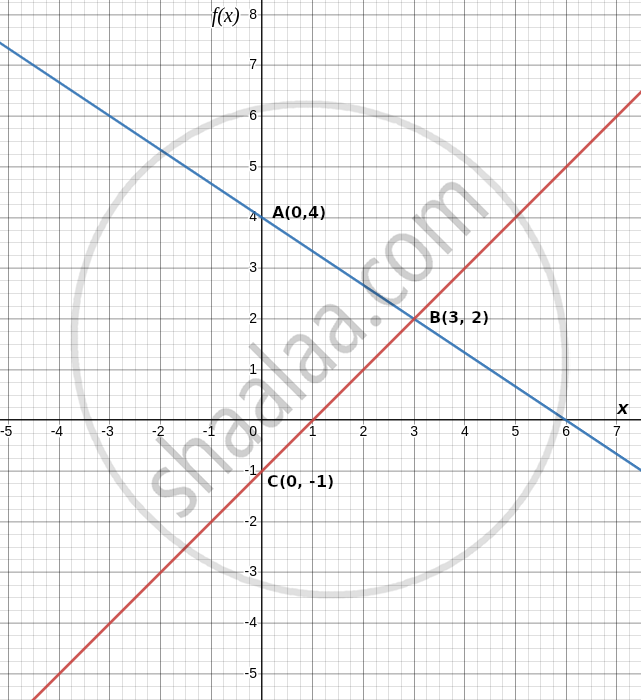# Draw the graphs of the following linear equations on the same graph paper. 2x + 3y = 12, x – y = 1.Find the co-ordinates of the vertices of the triangle formed by the two straight lines and the y-axis - Mathematics

Graph
Sum

Draw the graphs of the following linear equations on the same graph paper.
2x + 3y = 12, x – y = 1.
Find the co-ordinates of the vertices of the triangle formed by the two straight lines and the y-axis. Also, find the area of the triangle.

#### Solution

The given equations are:
2x + 3y = 12
x – y = 1

To graphically represent the above equations, we need at least two solutions for each equation.

For equation 2x + 3y = 12,

3y = 12 − 2x

y = (12 − 2x)/3

"If x = 0, then y"= [12 − 2(0)]/3 = [12 − 0]/3 = 12/3 = 4

"If x = 3, then y"= [12 − 2(3)]/3 = [12 − 6]/3 = 6/3 = 2

 x 0 3 y 4 2

For equation x − y = 1,

y = x − 1

If x = 0, then y = 0 − 1 = − 1

If x = 3, then y = 3 − 1 = 2

 x 0 3 y −1 2

The equation of y-axis is x = 0.

The following graph depicts the above situation:The lines AB, CD and AC represent the equations 2x + 3y = 12, x − y = 1 and y-axis respectively.

As we can see, the vertices of the given triangle are the points of intersection of the lines AB, CD, and AC taken in pairs.

We know that,

Area of ΔABC = 1/2 × Base × Height

The height of the triangle in the graph represents the distance between point B and the y-axis.

Height of the triangle = 3 units.

AC's base equals the distance between points A and C.

Base of the triangle = 4 + 1 = 5 units.

Area of the triangle formed by the given lines = 1/2 × 5 × 3 = 7.5 sq. units.

Concept: Graph of a Linear Equation in Two Variables
Is there an error in this question or solution?

#### APPEARS IN

RD Sharma Mathematics for Class 9
Chapter 7 Linear Equations in Two Variables
Exercise 7.3 | Q 17

Share